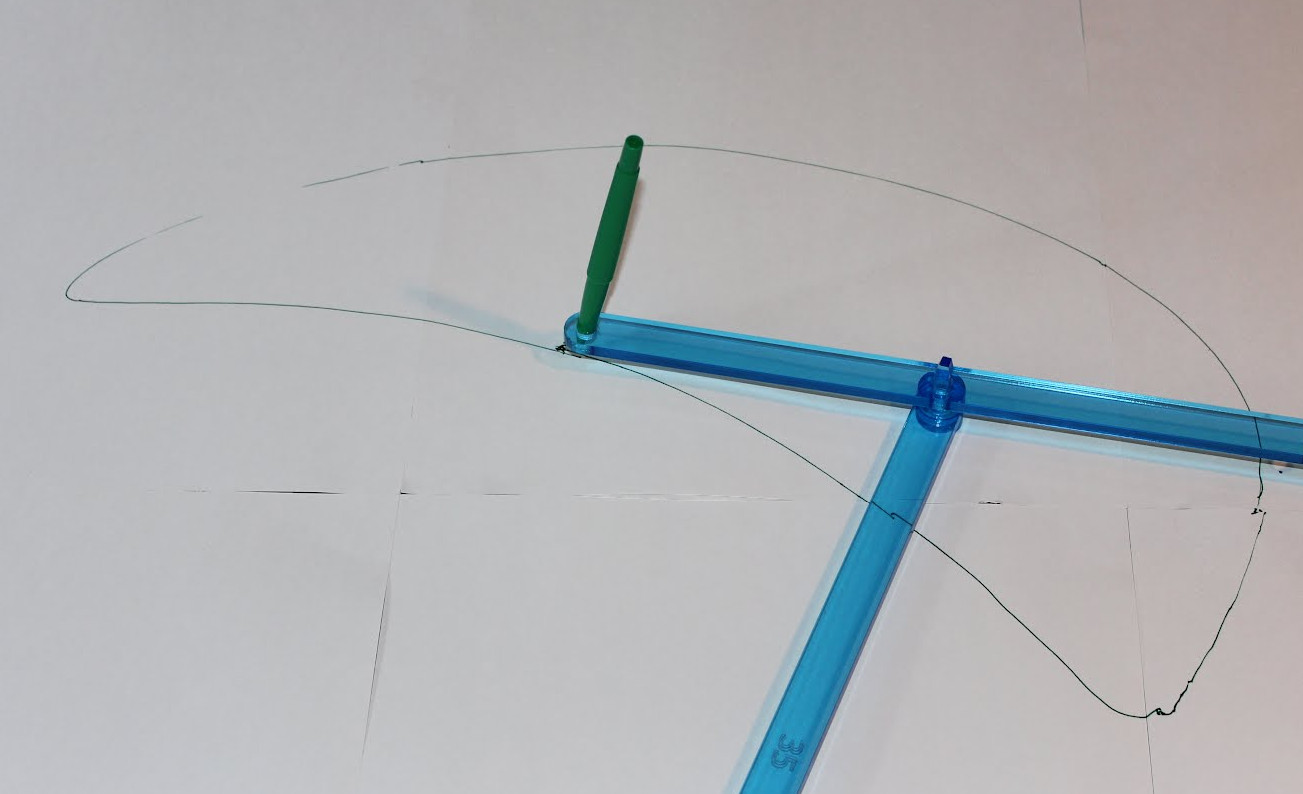Last time began a journey through the world of mechanical/mathematical linkages, a journey we take up in earnest today. See the previous column for the MoMath Linkage Kit, an introduction, and general instructions.

If we’re really going to understand linkages, what makes them tick, we should start from the very simplest. And one important way of measuring the complexity of a linkage is its number of “degrees of freedom”. This is basically the number of independent measurements you have to take of it at any given time to pin down exactly where every component is. You can also think of it as the number of “dimensions” in which the mechanism as a whole is able to move. For example, with the scissors jack as we used it last time, you could move the “input” pen up/down and left/right on the page, and that would determine exactly where every other component of the linkage had to be. (That’s why there was a cohesive, reproducible “output” drawing.) So that scissors jack had two degrees of freedom. Adding bars tends to increase the number of degrees of freedom; adding links tends to decrease it. (In fact, there’s a formula quantifying these phenomena, called the mobility formula.)

So, if we’re going to start simple, we should focus on linkages with one degree of freedom. (In fact, almost all of the linkages we’ll look in all have just one degree of freedom.) Another important measure of complexity is the number of bars. So, do we ever use a one-bar linkage? Absolutely: it’s called a compass. You fix one end, and use it to draw a circle.How about two-bar linkages? Well, if you fix just one joint, a two-bar linkage would have two degrees of freedom, and we’re not up to that yet. And if you fix an entire bar, then all that can happen is the other bar rotates, and you’ve got a compass on a tether…

So, on to three bars. If you fix one bar but don’t link the other two, you’ve got
siamese compasses that can draw two independent circles, not so interesting yet; and if you do link the other two, you have a triangle, which is rigid, i.e., has zero degrees of freedom — not quite enough.

Therefore, the simplest sort of linkage, other than the compass, is the four-bar linkage with one bar fixed, and the other four bars linked in a loop. But as it turns out, the world of four-bar linkages is already incredibly rich. To try to illustrate that, we’ll take a look today at recipe #2:

Complex 4-bar
Ingredients: A 50-rod (A), 25-rod (B), 60-rod with a 45-hole (C), a 35-rod (D), four linking sticks, and a pen.

Directions: Link A to B to C0; link C45 to D back to A. Put a pen in C60.How to use: Rotate bar B, one full turn. Note that at a few points you will have to “hop” one bar over another, because the linkage sticks protrude above and below the joints. Watch as the pen draws an unfamiliar curve:What is that curve? It’s certainly not a circle, or even an ellipse. Is it an area bounded by two circular arcs? Or an arc of a parabola? No and no. It looks a bit like a whale — maybe it’s the Moby Dick curve? Turns out, it’s a curve implicitly defined by a sixth-degree polynomial in x and y coordinates for the plane (as derived in Hartenberg & Danavit). In fact, a four-bar linkage is presumably the simplest apparatus you could make for drawing sextic curves! Next time, we’ll take a look at what sorts of things one can do with the flexibility afforded by that sixth degree…

More:
See all of our Math Monday columns